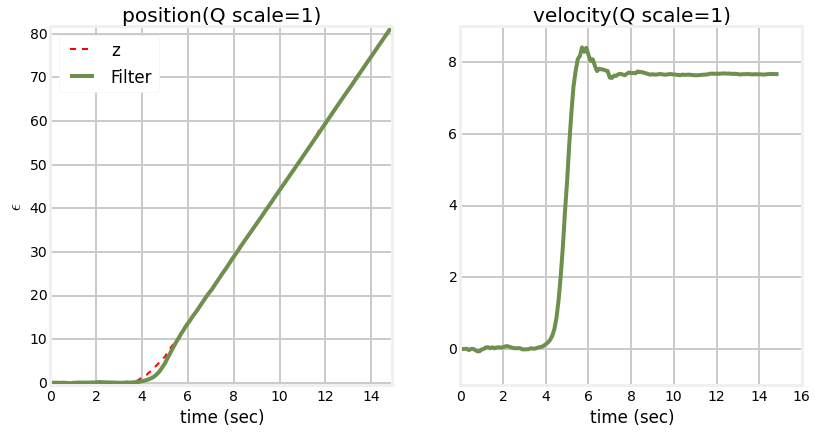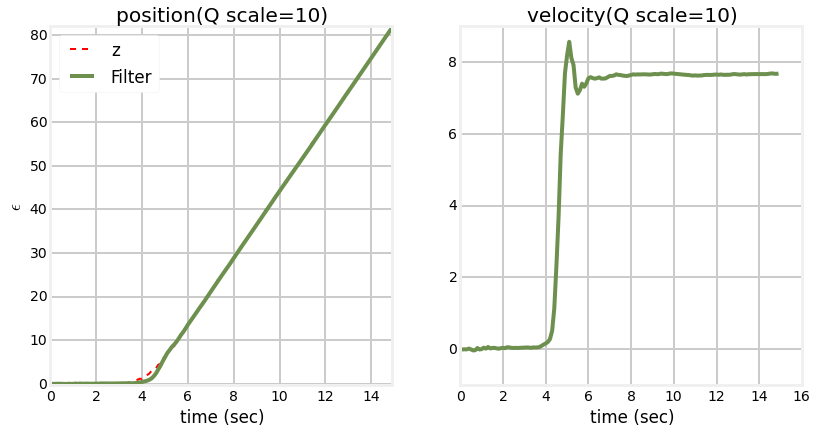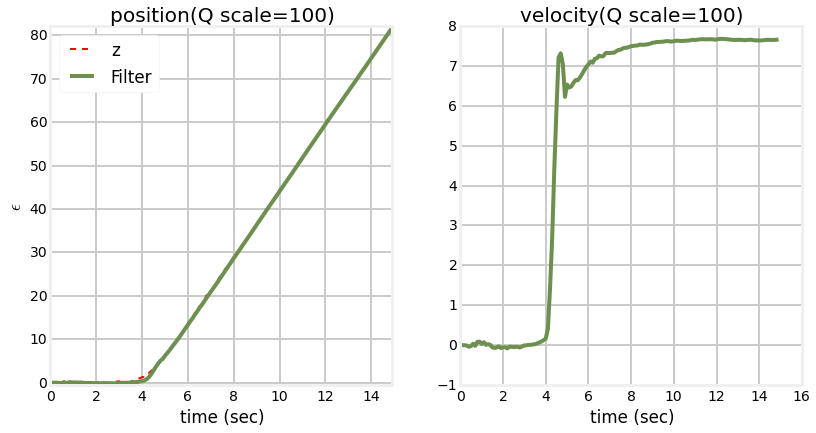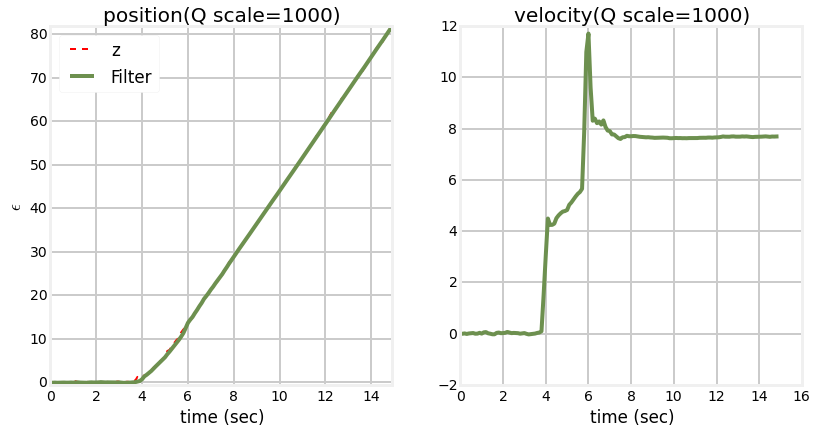In :
#format the book
%matplotlib inline
from __future__ import division, print_function
import matplotlib.pyplot as plt
import book_format

Out:

## Introduction¶

author's note: this chapter is in heavy development - read it if you want, but there are bugs in the sw, a lot of stuff if being revised, text may not match the plots, etc

So far we have considered the problem of tracking objects that are well behaved in relation to our process model. For example, we can use a constant velocity model track an object moving in a straight line. So long as the object moves in a straight line at a reasonably constant speed, or varies it's track and/or velocity very slowly this filter will perform very well. Suppose instead that we are trying to track a manuevering target, by which I mean an object with control inputs, such as a car along a road, an aircraft in flight, and so on. In these situations the filters perform quite poorly. Alternatively, consider a situation such as tracking a sailboat in the ocean. Even if we model the control inputs we have no way to model the wind or the ocean currents.

A first order approach to this problem is just to make the process noise $\mathbf{Q}$ larger to account for the unpredictability of the system dynamics. While this can work in the sense of providing a non-diverging filter, the result is typically far from optimal. The larger $\mathbf{Q}$ results in the filter giving more emphasis to the noise in the measurements. We will see an example of this shortly.

So in this chapter we will discuss the concept of an adaptive filter. This means about what it sounds like. The filter will adapt itself when it detects dynamics that the process model cannot account for. I will start with an example of the problem, and then discuss and implement various adaptive filters.

## Manuevering Targets¶

So let's begin by writing a simulation of a manuevering target. We are not interested in modeling anything with high fidelity, nor do we really care about 3 dimensions, so I will just implement a simple 2D model that you can provide steering inputs into. You can provide a new speed and/or direction, and it will modify its state to match.

In :
from math import sin, cos, radians

def angle_between(x,y):
return min(y-x, y-x+360, y-x-360, key=abs)

class ManueveringTarget(object):
def __init__(self, x0, y0, v0, heading):
self.x = x0
self.y = y0
self.vel = v0

self.cmd_vel = v0
self.vel_step = 0
self.hdg_step = 0
self.vel_delta = 0
self.hdg_delta = 0

def update(self):
self.x += vx
self.y += vy

if self.hdg_step > 0:
self.hdg_step -= 1
self.hdg += self.hdg_delta

if self.vel_step > 0:
self.vel_step -= 1
self.vel += self.vel_delta
return (self.x, self.y)

self.cmd_hdg = hdg_degrees
self.hdg_delta = angle_between(self.cmd_hdg, self.hdg) / steps
if abs(self.hdg_delta) > 0:
self.hdg_step = steps
else:
self.hdg_step = 0

def set_commanded_speed(self, speed, steps):
self.cmd_vel = speed
self.vel_delta = (self.cmd_vel - self.vel) / steps
if abs(self.vel_delta) > 0:
self.vel_step = steps
else:
self.vel_step = 0



Now let's implement a simulated sensor with noise.

In :
from numpy.random import randn

class NoisySensor(object):
def __init__(self, noise_factor=1):
self.noise_factor = noise_factor

def sense(self, pos):
return (pos + randn()*self.noise_factor,
pos + randn()*self.noise_factor)


Now let's generate a track and plot it to test that everything is working and to see what we will be trying to track. I'll put the data generation in a function so we can create paths of different lengths (why will be clear soon).

In :
from numpy import array
import book_plots as bp

noise_factor = 2.

t = ManueveringTarget(x0=0, y0=0, v0=0.3, heading=0)
xs = []
ys = []

for i in range(30):
x,y = t.update()
xs.append(x)
ys.append(y)

t.set_commanded_speed(1, 15)

x,y = t.update()
xs.append(x)
ys.append(y)

ns = NoisySensor(noise_factor=noise_factor)
pos = array(list(zip(xs, ys)))
zs = array([ns.sense(p) for p in pos])
return pos, zs

pos, zs = generate_data(50, noise_factor)
bp.plot_track(*zip(*pos))
plt.axis('equal')
plt.xlabel('X')
plt.ylabel('Y')
plt.title('2D Track')
plt.show()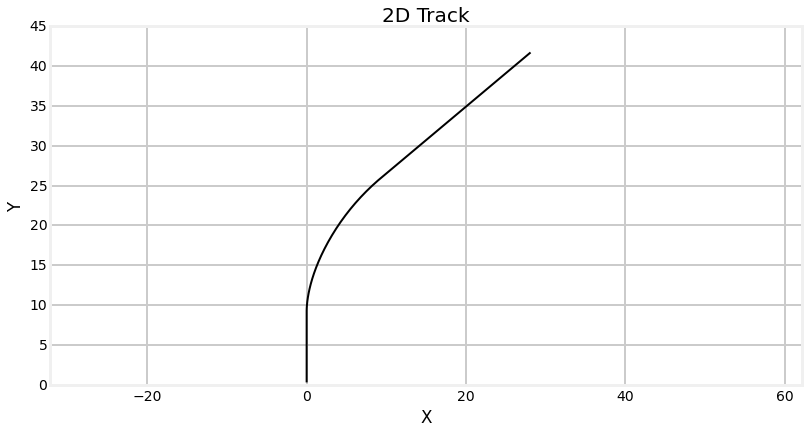And here is the track vs the simulated sensor readings.

In :
bp.plot_track(*zip(*pos))
bp.plot_measurements(*zip(*zs))
plt.axis('equal')
plt.legend(loc=4)
plt.xlabel('X')
plt.ylabel('Y')
plt.title('Track vs Measurements')
plt.show()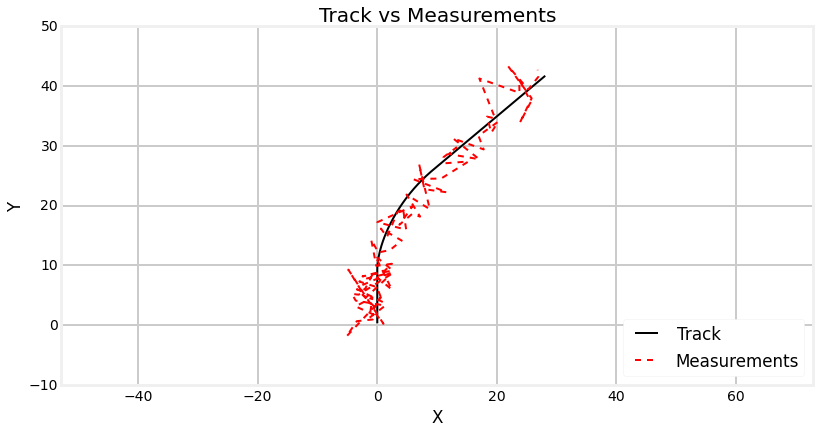This may appear to be an extreme amount of noise, but it will allow us to see the effect of various design choices more easily.

Now we can implement a Kalman filter to track this object. First let's implement a constant velocity filter. But let's make a simplification first. The x and y coordinates are independent, so we can track each independently. In the remainder of this chapter we will only track the x coordinate to keep the code and matrixes as small as possible.

In :
from filterpy.kalman import KalmanFilter
from filterpy.common import Q_discrete_white_noise

dt = 0.1
cvfilter = KalmanFilter(dim_x = 2, dim_z=1)
cvfilter.x = array([0., 0.])
cvfilter.P *= 3
cvfilter.R *= noise_factor
cvfilter.F = array([[1, dt],
[0,  1]], dtype=float)
cvfilter.H = array([[1, 0]], dtype=float)

cvfilter.Q = Q_discrete_white_noise(dim=2, dt=dt, var=0.02)

def initialize_filter(kf):
""" helper function - we will be reinitialing the filter
many times.
"""

kf.x.fill(0)
kf.P = np.eye(kf.x.shape) * .1


Just to be clear about the dimension reduction, if we wanted to track both x and y we would have written

cvfilter = KalmanFilter(dim_x = 4, dim_z=2)
cvfilter.x = array([0., 0., 0., 0.])
cvfilter.P *= 300
cvfilter.R *= 1
cvfilter.F = array([[1, dt, 0,  0],
[0,  1, 0,  0],
[0,  0, 1, dt],
[0,  0, 0,  1]], dtype=float)
cvfilter.H = array([[1, 0, 0, 0],
[0, 0, 1, 0]], dtype=float)

q = Q_discrete_white_noise(dim=2, dt=dt, var=0.020)
cvfilter.Q[0:2, 0:2] = q
cvfilter.Q[2:4, 2:4] = q
In :
# extract the x coordinates
import numpy as np

# initialize filter
initialize_filter(cvfilter)

xs = pos[:,0]
z_xs = zs[:,0]
# plot the results
kxs, _, _, _ = cvfilter.batch_filter(z_xs)
t = np.arange(0, len(z_xs) * dt, dt)
bp.plot_track(t, xs)
bp.plot_filter(t, kxs[:,0], label='KF')
plt.xlabel('time (sec)')
plt.ylabel('X')
plt.legend(loc=4)
plt.title('Track vs KF')
plt.show()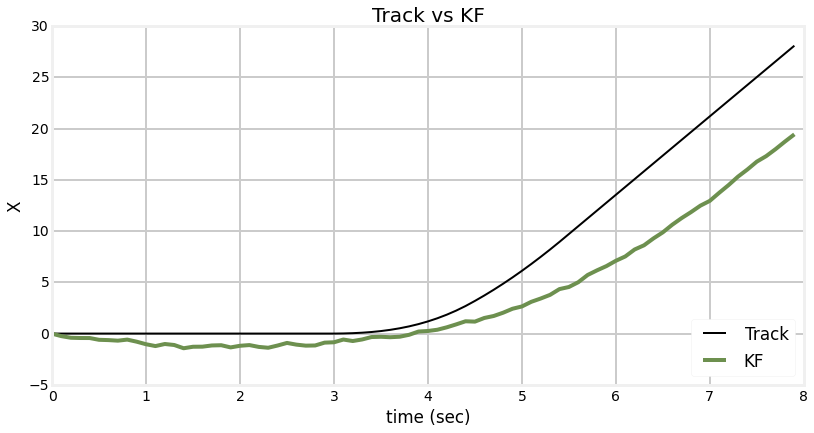We can see from the plot that the Kalman filter was unable to track the change in heading. Recall from the g-h Filter chapter that this is because the filter is not modelling acceleration, hence it will always lag the input. The filter will eventually catch up with the signal if the signal enters a steady state. Let's look at that.

In :
# reinitialize filter
initialize_filter(cvfilter)

pos2, zs2 = generate_data(120, noise_factor)
xs2 = pos2[:,0]
z_xs2 = zs2[:,0]
t = np.arange(0, len(xs2) * dt, dt)
# plot the results
kxs2, _, _, _ = cvfilter.batch_filter(z_xs2)

bp.plot_track(t, xs2)
bp.plot_filter(t, kxs2[:,0], label='KF')
plt.xlabel('time (sec)')
plt.ylabel('X')
plt.title('Effects of Acceleration')
plt.legend(loc=4)
plt.show()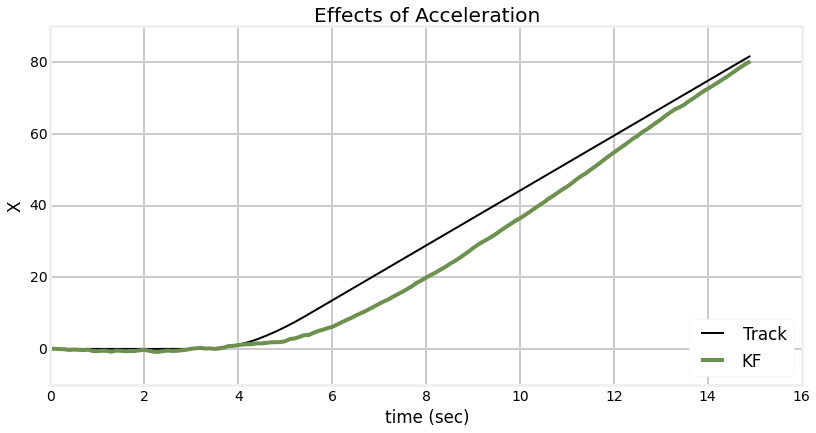The underlying problem is that our process model is correct for the steady state sections, but incorrect for when the object is manuevering. We can try to account for this by increasing the size of Q, like so.

In :
# reinitialize filter
initialize_filter(cvfilter)
cvfilter.Q = Q_discrete_white_noise(dim=2, dt=dt, var=2.0) # var was 0.02

# recompute track
kxs2, _, _, _ = cvfilter.batch_filter(z_xs2)

bp.plot_track(t, xs2)
bp.plot_filter(t, kxs2[:,0], label='KF')
plt.legend(loc=4)
plt.xlabel('time (sec)')
plt.ylabel('X')
plt.title('Large Q (var=2.0)')
plt.show()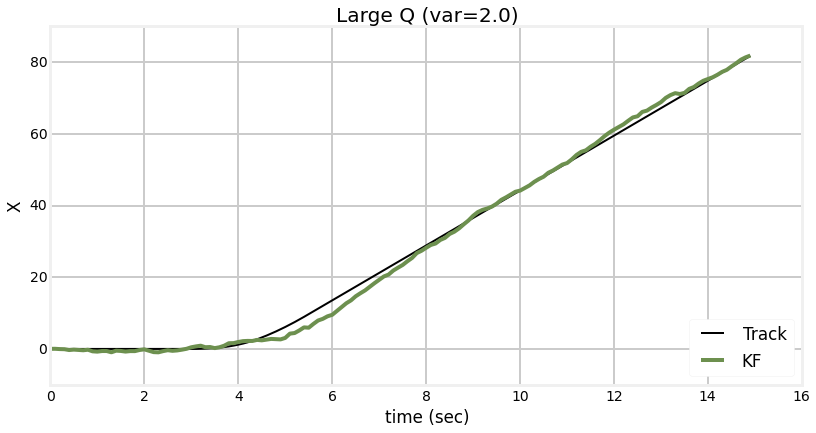We can see that the filter reacquired the track more quickly, but at the cost of a lot of noise in the output. Furthermore, many tracking situations could not tolerate the amount of lag shown between seconds 4 and 8. We could reduce it further at the cost of very noisy output, like so:

In :
# reinitialize filter
initialize_filter(cvfilter)
cvfilter.Q = Q_discrete_white_noise(dim=2, dt=dt, var=20.0) # var was 0.02

# recompute track
cvfilter.x = array([0., 0.])
kxs2, _, _, _ = cvfilter.batch_filter(z_xs2)

bp.plot_track(t, xs2)
bp.plot_filter(t, kxs2[:,0], label='KF')
plt.legend(loc=4)
plt.xlabel('time (sec)')
plt.ylabel('X')
plt.title('Huge Q (var=20.0)')
plt.show()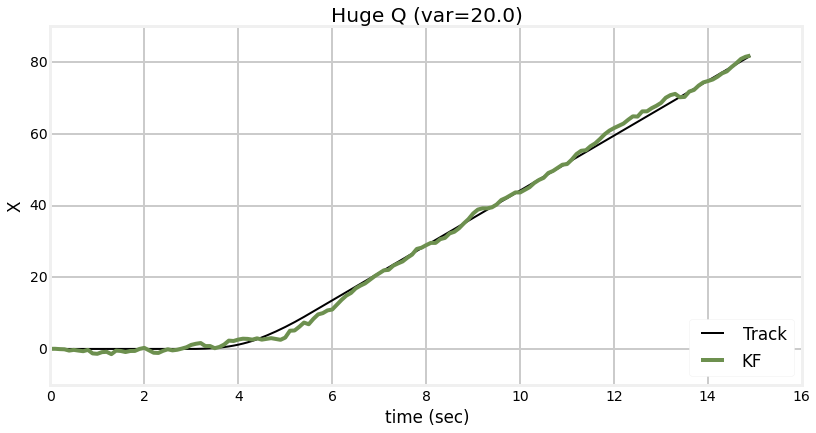Manuevers imply acceleration, so let's implement a constant acceleration Kalman filter and see how it fairs with the same data.

In :
cafilter = KalmanFilter(dim_x = 3, dim_z=1)
cafilter.x = array([0., 0., 0.])
cafilter.P *= 3
cafilter.R *= 1
cafilter.F = array([[1, dt, 0.5*dt*dt],
[0, 1,         dt],
[0, 0,          1]], dtype=float)
cafilter.H = array([[1, 0, 0]], dtype=float)

def initialize_const_accel(f):
f.x = array([0., 0., 0.])
f.P = np.eye(3) * 3

cafilter.Q = Q_discrete_white_noise(dim=3, dt=dt, var=0.02)

In :
initialize_const_accel(cafilter)

kxs2, _, _, _ = cafilter.batch_filter(z_xs2)
bp.plot_track(t, xs2)
bp.plot_filter(t, kxs2[:,0], label='KF')
plt.legend(loc=4)
plt.xlabel('time (sec)')
plt.ylabel('X')
plt.title('Constant Acceration Kalman Filter')
plt.show()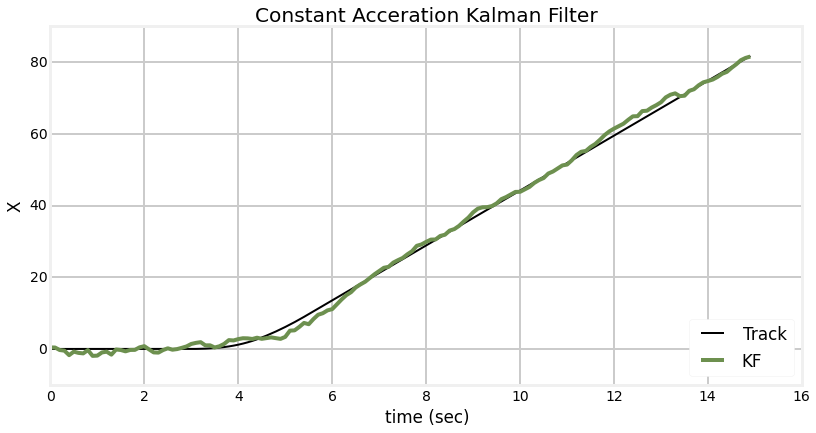The constant acceleration model is able to track the manuever with no lag, but at the cost of very noisy output during the steady state behavior. The noisy output is due to the filter being unable to distinguish between the beginning of an manuever and just noise in the signal. Noise in the signal implies an acceleration, and so the acceleration term of the filter tracks it.

It seems we cannot win. A constant velocity filter cannot react quickly when the target is accelerating, but a constant acceleration filter misinterprets noise during zero acceleration regimes as legitimate acceleration.

Yet there is an important insight here that will lead us to a solution. When the target is not manuevering (the acceleration is zero) the constant velocity filter performs optimally. When the target is manuevering the constant acceleration filter performs well, as does the constant velocity filter with an artificially large process noise $\mathbf{Q}$. If we make a filter that adapts itself to the behavior of the tracked object we could have the best of both worlds.

## Detecting a Manuever¶

Before we discuss how to create an adaptive filter we have to ask how do we detect a manuever? We cannot reasonably adapt a filter to respond to manuevers if we do not know when a manuever is happening.

We have been defining manuever as the time when the tracked object is accelerating, but in general we can say that the object is manuevering with respect to the Kalman filter if its behavior is different than the process model being used by the filter.

What is the mathematical consequence of a manuevering object for the filter? The object will be behaving differently than predicted by the filter, so the residual will be large. Recall that the residual is the difference between the current prediction of the filter and the measurement.

In :
from mkf_internal import show_residual_chart
show_residual_chart()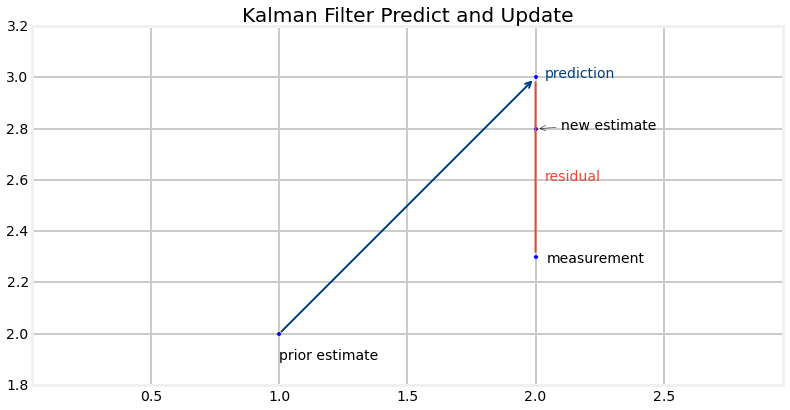To confirm this, let's plot the residual for the filter during the manuever. I will reduce the amount of noise in the data to make it easier to see the residual.

In :
pos2, zs2 = generate_data(120, noise_factor=0.2)
xs2 = pos2[:,0]
z_xs2 = zs2[:,0]

# reinitialize filter
initialize_filter(cvfilter)
cvfilter.Q = Q_discrete_white_noise(dim=2, dt=dt, var=0.02)
xs = []
res = []
for z in z_xs2:
cvfilter.predict()
cvfilter.update([z])
xs.append(cvfilter.x)
res.append(cvfilter.y)
xs = np.asarray(xs)
plt.subplot(121)
bp.plot_measurements(t, z_xs2, label='z')
bp.plot_filter(t, xs)
plt.legend(loc=2)
plt.xlabel('time (sec)')
plt.ylabel('X')
plt.title('estimates vs measurements')
plt.subplot(122)
# plot twice so it has the same color as the plot to the left!
plt.plot(t, res)
plt.plot(t, res)
plt.xlabel('time (sec)')
plt.ylabel('residual')
plt.title('residuals')
plt.show()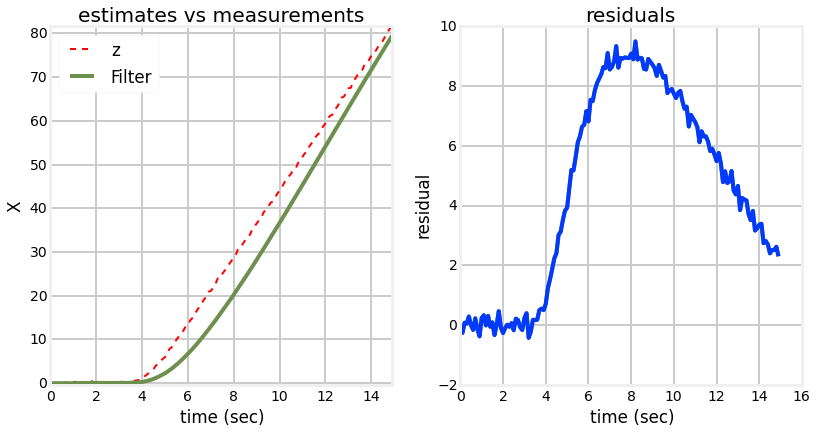On the left I have plotted the noisy measurements (not the track!) against the Kalman filter output. On the right I display the residuals computed by the filter - the difference between the measurement and the predictions made by the Kalman filter. Let me emphasize this to make this clear. The plot on the right is not merely the difference between the two lines in the left plot. The left plot shows the difference between the measurements and the final Kalman filter output, whereas the right plot shows us the difference between the plot and the predictions of the process model.

That may seem like a subtle distinction, but from the plots you see it is not. The amount of deviation in the left plot when the manuever starts is small, but the deviation in the right tells a very different story. If the tracked object was moving according to the process model the residual plot should bounce around 0.0. This is because the measurements will be obeying the equation

$$measurement = process\_model(t) + noise(t)$$

.

Once the target starts manuevering the predictions of the target behavior will not match the behavior as the equation will be

$$measurement = process\_model(t) + manuever\_delta(t) + noise(t)$$

.

Therefore if the residuals diverge from a mean of 0.0 we know that a manuever has commenced.

We can see from the residual plot that we have our work cut out for us. We can clearly see the result of the manuever in the residual plot, but the amount of noise in the signal obscures the start of the manuever. This is our age old problem of extracting the signal from the noise.

The first approach we will consider will use a lower order model and adjust the process noise based on whether a manuever is occuring or not. When the residual gets large (for some reasonalbe definition of large) we will increase the process noise. This will cause the filter to favor the measurement over the process prediction and the filter will track the signal closely. When the residual is small we will then scale back the process noise.

There are many ways of doing this in the literature, I will consider a couple of choices.

The first method (from Bar-Shalom ) normalizes the square of the residual using the following equation:

$$\epsilon = \mathbf{y^\mathsf{T}S}^{-1}\mathbf{y}$$

where $\mathbf{y}$ is the residual and $\mathbf{S}$ is the measurement covariance, which has the equation

$$\mathbf{S} = \mathbf{HPH^\mathsf{T}} + \mathbf{R}$$

If the linear algebra used to compute this confuses you, recall that we can think of matrix inverses as division, so $\epsilon = \mathbf{y^\mathsf{T}S}^{-1}\mathbf{y}$ can be thought of as computing

$$\epsilon=\frac{\mathbf{y}^2}{\mathbf{S}}$$

Both $\mathbf{y}$ and $\mathbf{S}$ are attributes of filterpy.KalmanFilter so implementation is of this computation will be straightforward.

Let's look at a plot of $\epsilon$ against time.

In :
from filterpy.common import dot3
from numpy.linalg import inv

# reinitialize filter
initialize_filter(cvfilter)

cvfilter.R = np.eye(1)*0.2
cvfilter.Q = Q_discrete_white_noise(dim=2, dt=dt, var=0.02)
epss = []
for z in z_xs2:
cvfilter.predict()
cvfilter.update([z])
y = cvfilter.y
S = cvfilter.S
eps = dot3(y.T, inv(cvfilter.S), y)
epss.append(eps)

plt.plot(t, epss)
plt.ylabel('$\epsilon$')
plt.xlabel('time (sec)')
plt.title('Epsilon vs time')
plt.show()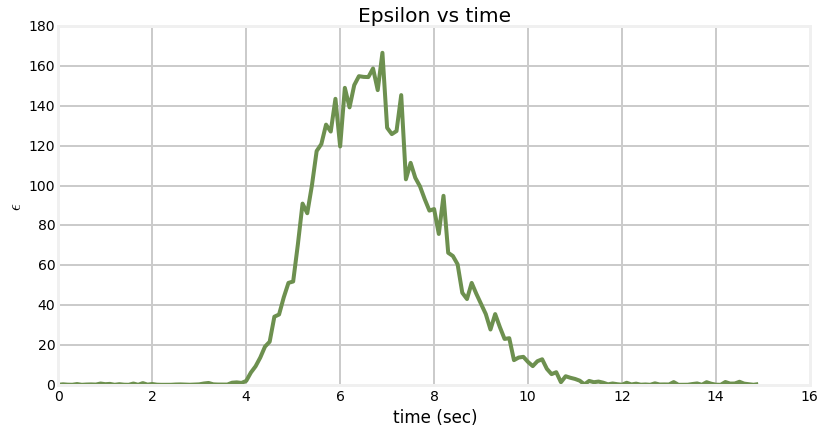This plot should make clear the effect of normalizing the residual. Squaring the residual ensures that the signal is always greater than zero, and normalizing by the meausement covariance scales the signal so that we can distinguish when the residual is markedly changed relative to the measurement noise. The manuever starts at t=3 seconds, and we can see that $\epsilon$ starts to increase rapidly not long after that.

We will want to start scaling $\mathbf{Q}$ up once $\epsilon$ exceeds some limit, and back down once it again falls below that limit. We multiply $\mathbf{Q}$ by a scaling factor. Perhaps there is literature on choosing this factor analytically; I just derive it experimentally. We can be somewhat more analytical about choosing the limit for $\epsilon$ (named $\epsilon_{max}$) - generally speaking once the residual is greater than 3 standard deviations or so we can assume the difference is due to a real change and not to noise. However, sensors are rarely truly Gaussian and so a larger number, such as 5-6 standard deviations is used in practice.

I have implemented this algorithm using reasonable values for $\epsilon_{max}$ and the $\mathbf{Q}$ scaling factor. To make inspection of the result easier I have limited the plot to the first 10 seconds of simulation.

In :
from filterpy.common import dot3
from numpy.linalg import inv

# reinitialize filter
initialize_filter(cvfilter)
cvfilter.R = np.eye(1)*0.2

cvfilter.Q = Q_discrete_white_noise(dim=2, dt=dt, var=0.02)

Q_scale_factor = 1000.
eps_max = 4.

epss = []
xs = []
count = 0
for i,z in zip(t,z_xs2):
cvfilter.predict()
cvfilter.update([z])
y = cvfilter.y
S = cvfilter.S
eps = dot3(y.T, inv(cvfilter.S), y)
epss.append(eps)
xs.append(cvfilter.x)

if eps > eps_max:
cvfilter.Q *= Q_scale_factor
count += 1
elif count > 0:
cvfilter.Q /= Q_scale_factor
count -= 1

bp.plot_measurements(t, z_xs2, lw=6, label='z')
bp.plot_filter(t, xs, label='filter')
plt.ylabel('$\epsilon$')
plt.xlabel('time (sec)')
plt.legend(loc=4)
plt.title('epsilon=4')
plt.show()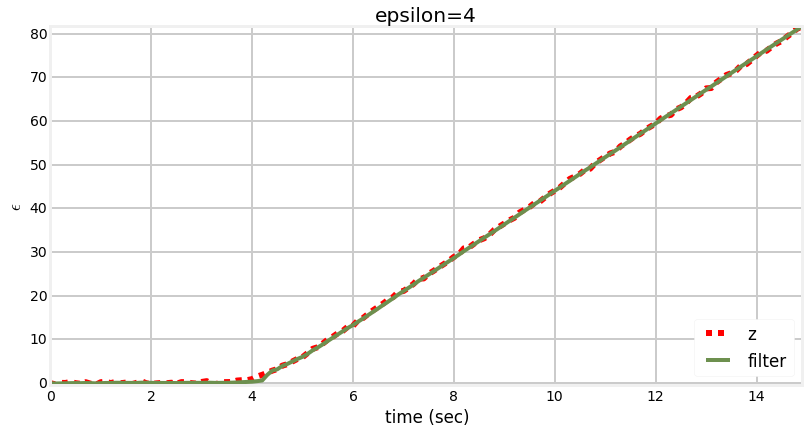The performance of this filter is markedly better than the constant velocity filter. The constant velocity filter took roughly 10 seconds to reacquire the signal after the start of the manuever. The adaptive filter takes under a second to do the same.

### Continuous Adjustment - Standard Deviation Version¶

Another, very similar method from Zarchan  sets the limit based on the standard deviation of the measurement error covariance. Here the equations are:

\begin{aligned} std &= \sqrt{\mathbf{HPH}^\mathsf{T} + \mathbf{R}} \\ &= \sqrt{\mathbf{S}} \end{aligned}

If the absolute value of the residual is more than some multiple of the standard deviation computed above we increase the process noise by a fixed amount, recompute Q, and continue.

In :
from filterpy.common import dot3
from numpy.linalg import inv
from math import sqrt

std_title=False,
Q_title=False):
pos2, zs2 = generate_data(120, noise_factor=0.2)
xs2 = pos2[:,0]
z_xs2 = zs2[:,0]

# reinitialize filter
initialize_filter(cvfilter)

cvfilter.R = np.eye(1)*0.2

phi = 0.02
cvfilter.Q = Q_discrete_white_noise(dim=2, dt=dt, var=phi)
xs = []
ys = []
count = 0
for i,z in zip(t,z_xs2):
cvfilter.predict()
cvfilter.update([z])
y = cvfilter.y
S = cvfilter.S
std = sqrt(S)

xs.append(cvfilter.x)
ys.append(y)

if abs(y) > std_scale*std:
phi += Q_scale_factor
cvfilter.Q = Q_discrete_white_noise(dim=2, dt=dt, var=phi)
count += 1
elif count > 0:
phi -= Q_scale_factor
cvfilter.Q = Q_discrete_white_noise(dim=2, dt=dt, var=phi)
count -= 1

xs = np.asarray(xs)
plt.subplot(121)
bp.plot_measurements(t, z_xs2, label='z')
bp.plot_filter(t, xs[:,0])
plt.ylabel('$\epsilon$')
plt.xlabel('time (sec)')
plt.legend(loc=2)
if std_title:
plt.title('position(std={})'.format(std_scale))
elif Q_title:
plt.title('position(Q scale={})'.format(Q_scale_factor))
else:
plt.title('position')

plt.subplot(122)
plt.plot(t, xs[:,1])
plt.xlabel('time (sec)')
if std_title:
plt.title('velocity(std={})'.format(std_scale))
elif Q_title:
plt.title('velocity(Q scale={})'.format(Q_scale_factor))
else:
plt.title('velocity')

plt.show()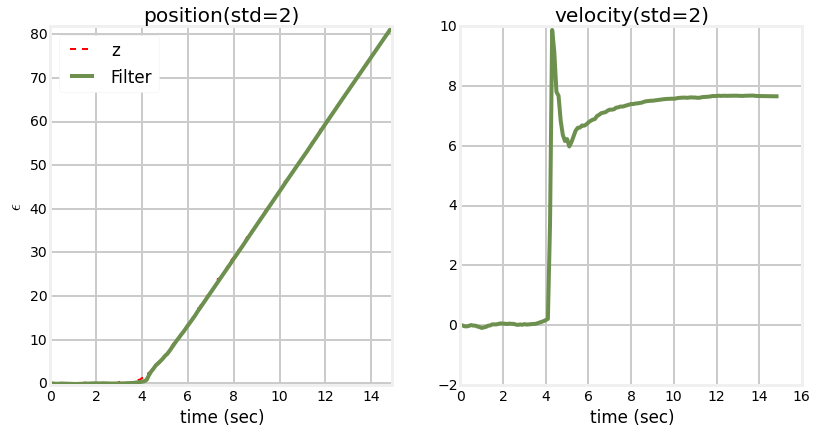So I chose to use 1000 as the scaling factor for the noise, and 2 as the standard deviation limit. Why these numbers? Well, first, let's look at the difference between 2 and 3 standard deviations.

Two Standard Deviations

In :
zarchan_adaptive_filter(1000, 2, std_title=True)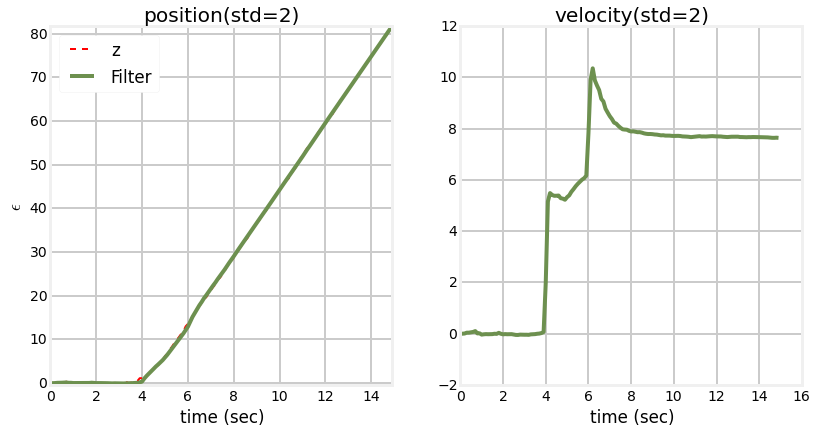Three Standard Deviations

In :
zarchan_adaptive_filter(1000, 3, std_title=True)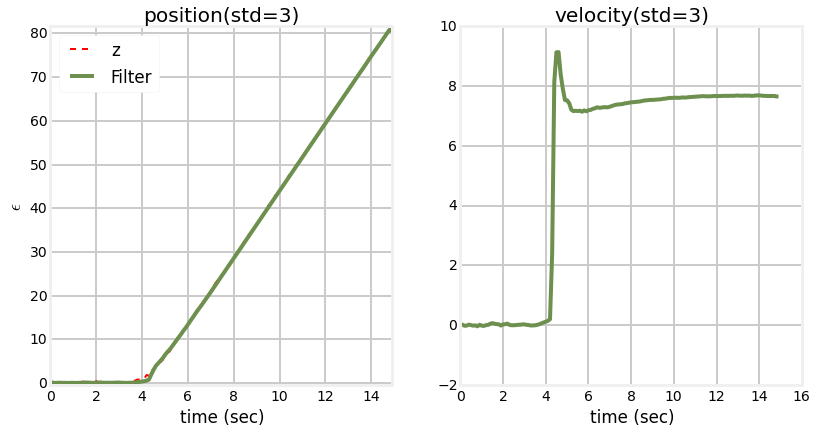We can see from the charts that the filter output for the position is very similar regardless of weather we use 2 standard deviations or three. But the computation of the velocity is a different matter. Let's explore this further. First, lets make the standard deviation very small.

In :
zarchan_adaptive_filter(1000, .1, std_title=True)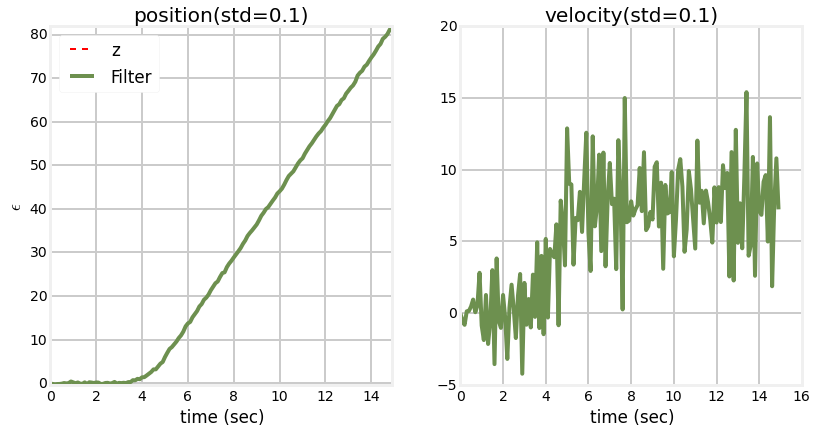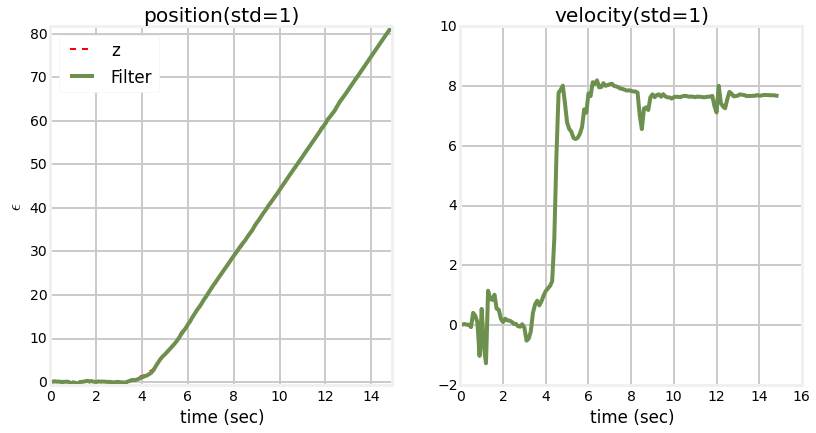As the standard deviation limit gets smaller the computation of the velocity gets worse. Think about why this is so. If we start varying the filter so that it perfers the measurement over the prediction as soon as the residual deviates even slightly from the prediction we very quickly be giving almost all the weight towards the measurment. With no weight for the prediction we have no information from which to create the unobserved variables. So, when the limit is 0.1 std you can see that the velocity is swamped by the noise in the measurement. On the other hand, because we are favoring the measurements so much the position follows the manuver almost perfectly.

Now let's look at the effect of various increments for the process noise. Here I have held the standard deviation limit to 2 std, and varied the increment from 1 to 10,000.

In :
zarchan_adaptive_filter(1, 2, Q_title=True)